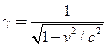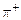Chapter 33, Problem 46PE

Chapter
Section
Textbook Problem

Integrated ConceptsCalculate the relativistic quantityfor 1.00−TeV protons produced at Fermilab. (b) If such a proton created ahaving the same speed, how long would its life be in the laboratory? (c) How far could it travel in this time?

To determine

(a)

The relativistic quantity for 1 TeV protons produced at fermi lab.

Explanation

Given:

Energy of protons produced at fermi lab =1 TeV

Formula used:

Total energy of any relativistic particle, E=γE0

Where, γ relativistic quantity

E0 rest energy of the particle

Calculation:

Rest energy of proton, E0=938 MeV

Therefore, relativistic quantity for 1 TeV protons,

To determine

(b)

The life time of π+ particle created by 1 TeV protons in the laboratory with the same speed

as that of protons.

To determine

(c)

The distance travelled π+ particle created by 1 TeV protons in the laboratory with the same

speed as that of protons.

Still sussing out bartleby?

Check out a sample textbook solution.

See a sample solution

The Solution to Your Study Problems

Bartleby provides explanations to thousands of textbook problems written by our experts, many with advanced degrees!

Get Started